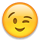Is it possible to filter an instance on a circular shape ?

Hello,
I want to remove instances which do not overlap with a circular form using a Python script.
For sure, Klayout gives the possibility to check the overlap betwwen two boxes, but I don't see how to check the overlap between a box and a circular form.

box=....
if not box.overlaps(db.Box(inst.bbox())):
inst.delete()

Thank you.

Regards,

• @ahmedo

This will find all boxes outside of a layer/circle
Run the script and you will see a circle with boxes inside,
click undo and you can see what has been removed

# Enter your Python code here import pya import array import sys import math import time mw = pya.Application.instance().main_window() layout = pya.Application.instance().main_window().current_view().active_cellview().layout() topcell = pya.Application.instance().main_window().current_view().active_cellview().cell lv = pya.Application.instance().main_window().current_view() if layout == None: raise Exception("No layout") cv = pya.Application.instance().main_window().current_view().active_cellview() dbu =layout.dbu cell = pya.Application.instance().main_window().current_view().active_cellview().cell if cell == None: raise Exception("No cell") n = 2000 # number of points r = 2*2 # radius da = 2 * math.pi / n def coords(path): result = [] for p in path: result.append(DPoint.new(p/dbu,p/dbu)) return result def create_polygon(crd,lay): layer_info = LayerInfo.new(lay,0) layer_index = layout.layer(layer_info) cv.cell.shapes(layer_index).insert(Polygon.new(crd)) def create_box(crd,lay): layer_info = LayerInfo.new(lay,0) layer_index = layout.layer(layer_info) cv.cell.shapes(layer_index).insert(Box.new(crd,crd)) circle = [] for i in range(0, n): path = r * math.cos(i * da), r * math.sin(i * da) circle.append(path) lay1 = 9 lay2 = 5 pth = coords(circle) create_polygon(pth,lay1) box = [(-6.818,-1.185), (-5.195,3.591)] crd = coords(box) create_box(crd,lay2) box = [(5.742,-2.333), (7.674,2.771)] crd = coords(box) create_box(crd,lay2) box = [(-2.827,0.849), (-1.326,2.469)] crd = coords(box) create_box(crd,lay2) box = [(0.730,-2.708), (2.231,-1.088)] crd = coords(box) create_box(crd,lay2) def cut_region(lay1,lay2): lv.transaction("Undo") lif1 = LayerInfo.new(lay1,0) li1 = layout.layer(lif1) lif2 = LayerInfo.new(lay2,0) li2 = layout.layer(lif2) r1 = pya.Region(topcell.begin_shapes_rec(li1)) r2 = pya.Region(topcell.begin_shapes_rec(li2)) #Boolean Functions #outside = r1 - r2 #outside = r2 - r1 #outside = r2 + r1 #outside = r1 + r2 #outside = r1 & r2 outside = r2 & r1 #outside = r2 ^ r1 #outside = r1 ^ r2 layout.clear_layer(li2) output = layout.layer(lif2) topcell.shapes(output).insert(outside) lay1 = 9 lay2 = 5 cut_region(lay1,lay2) lv.commit()
• If I understand @ahmedo correctly he said not overlapping, so instead of
outside = r2 & r1
it should be
not_outside = r2.select_not_outside(r1)
and then
topcell.shapes(output).insert(not_outside)

r1 & r2 will cut parts of boxes that are outside of the circle.

• @sebastian

I may have misread .. but good point and thanks for highlighting this, we all learn

• No worries ^^. I also think overlapping is not clearly defined in daily speech, so you may very well be correct. Regions were confusing when I started with KLayout, so I wanted to point out that there are fancy options in the regions included that might save a lot of time.

• Thank you @tagger5896 and @sebastian for your valuable help.

I will make a test with your code. I see that the code looks for the name of the layout at the beginning, so I guess I need to run the script using the command line:
klayout -r <script.py> my.gds

Is it right ?

By the way I have implemented a solution that allows me to check the overlap:

filter_poly = db.Polygon(circular_shape.polygon)
for inst in layout_insts:
# Define the 4 points of the instance box
llp = db.Point(inst.bbox().left,inst.bbox().bottom)
lrp = db.Point(inst.bbox().right,inst.bbox().bottom)
tlp = db.Point(inst.bbox().left,inst.bbox().top)
trp = db.Point(inst.bbox().right,inst.bbox().top)

let = 0
if filter_poly.inside(llp) or filter_poly.inside(lrp) or filter_poly.inside(tlp) or filter_poly.inside(trp):
let = 1

# if let = 0 , then remove instance.
if let == 0 :
inst.delete()

Regards

• For my question I think that I have undestood that @tagger5896 's code should be executed from macro menuI'm used to creating scripts that I execute always in batch mode.

Regards

• @ahmedo
You should have an open layout and run the code from the Macro IDE menu yes.

• @tagger5896

Thank you so much for your help.

Regards

• @sebastian
thanks for pointing this out ... floodgates open and the sea's part,
and yes this makes it easiernot_outside = r2.select_not_outside(r1)